Back to Adaptive Vision Studio website

You are here: Start » Filter Reference » Logic

Logic

Select a filter from the list below.

IconNameDescription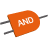AndReturns True if (and only if) all arguments are True.And_OfArrayReturns True if (and only if) all values in the input array True.And_OfLoopReturns True if (and only if) all values appearing in consecutive iterations are True.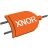IffReturns True if (and only if) the input arguments are both True or both False.MergeAndReturns True if (and only if) all arguments are True. Accepts conditional values.MergeAnd_OfArrayReturns True if (and only if) all values in the input array True. Accepts conditional values.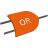MergeOrReturns True if (and only if) any of the arguments is True. Accepts conditional values.MergeOr_OfArrayReturns True if (and only if) at least one value in the input array is True. Accepts conditional values.NotReturns True if (and only if) the input argument is False.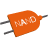NotAndReturns True if (and only if) at least one of the arguments is False.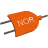NotOrReturns True if (and only if) both arguments are False.OrReturns True if (and only if) any of the arguments is True.Or_OfArrayReturns True if (and only if) at least one value in the input array is True.Or_OfLoopReturns True if (and only if) at least one value appearing in consecutive iterations is True.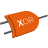XorReturns True if (and only if) the number of True input values is odd.Xor_OfArrayReturns True if (and only if) the number of True values in the input array is odd.Xor_OfLoopReturns True if (and only if) the number of True values appearing in consecutive iterations is odd.